# Functions 1 1162022 2 1162022 Advantages Program writing

• Slides: 29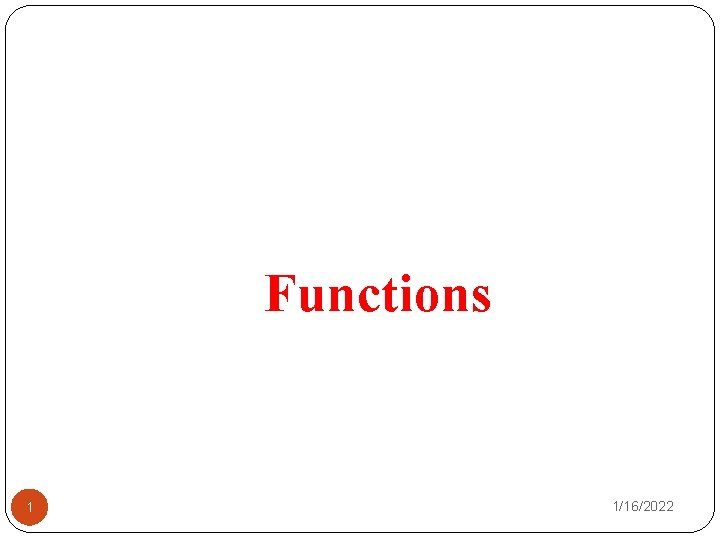Functions 1 1/16/2022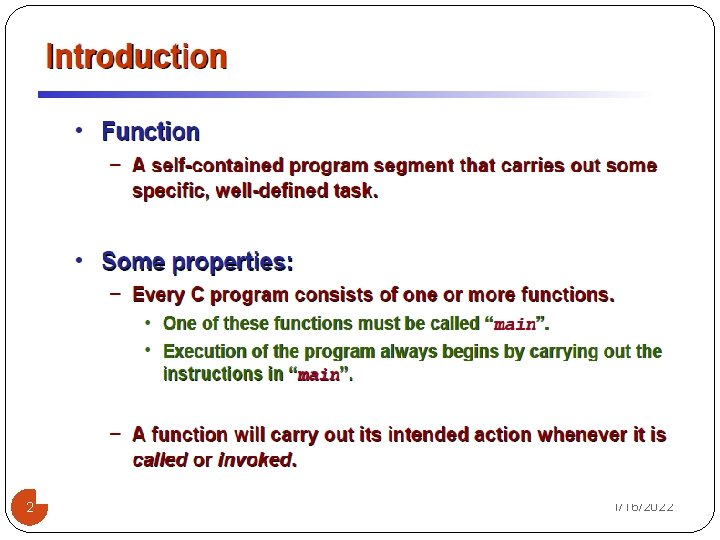2 1/16/2022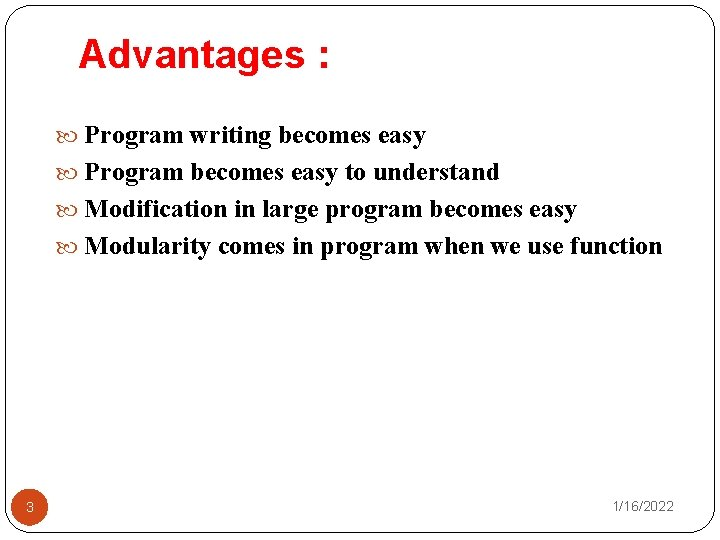Advantages : Program writing becomes easy Program becomes easy to understand Modification in large program becomes easy Modularity comes in program when we use function 3 1/16/2022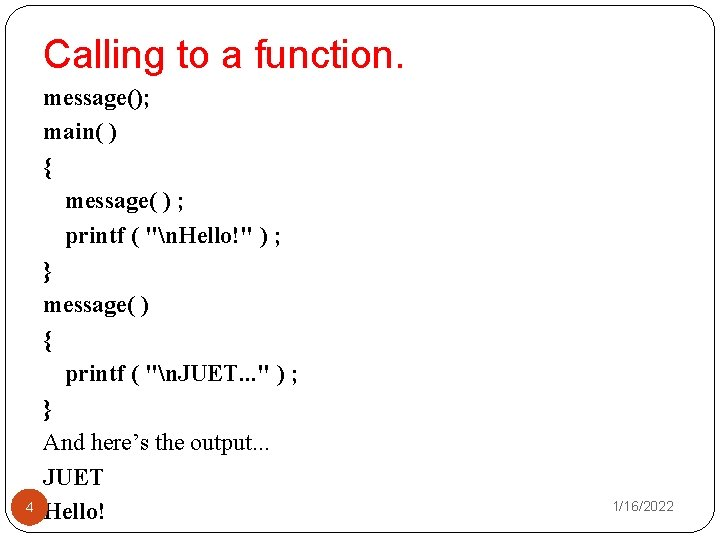Calling to a function. 4 message(); main( ) { message( ) ; printf ( "n. Hello!" ) ; } message( ) { printf ( "n. JUET. . . " ) ; } And here’s the output. . . JUET Hello! 1/16/2022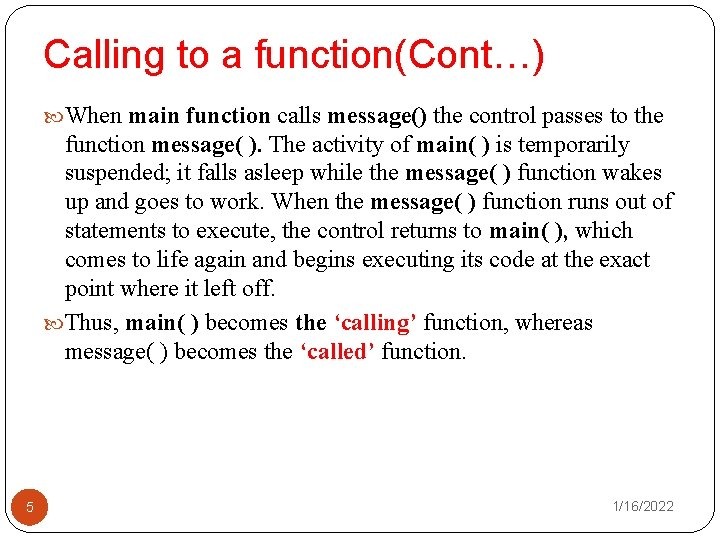Calling to a function(Cont…) When main function calls message() the control passes to the function message( ). The activity of main( ) is temporarily suspended; it falls asleep while the message( ) function wakes up and goes to work. When the message( ) function runs out of statements to execute, the control returns to main( ), which comes to life again and begins executing its code at the exact point where it left off. Thus, main( ) becomes the ‘calling’ function, whereas message( ) becomes the ‘called’ function. 5 1/16/2022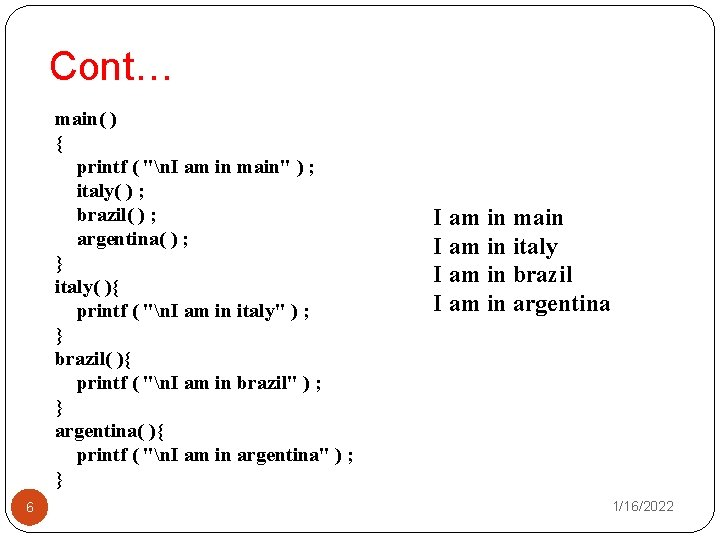Cont… main( ) { printf ( "n. I am in main" ) ; italy( ) ; brazil( ) ; argentina( ) ; } italy( ){ printf ( "n. I am in italy" ) ; } brazil( ){ printf ( "n. I am in brazil" ) ; } argentina( ){ printf ( "n. I am in argentina" ) ; } 6 I am in main I am in italy I am in brazil I am in argentina 1/16/2022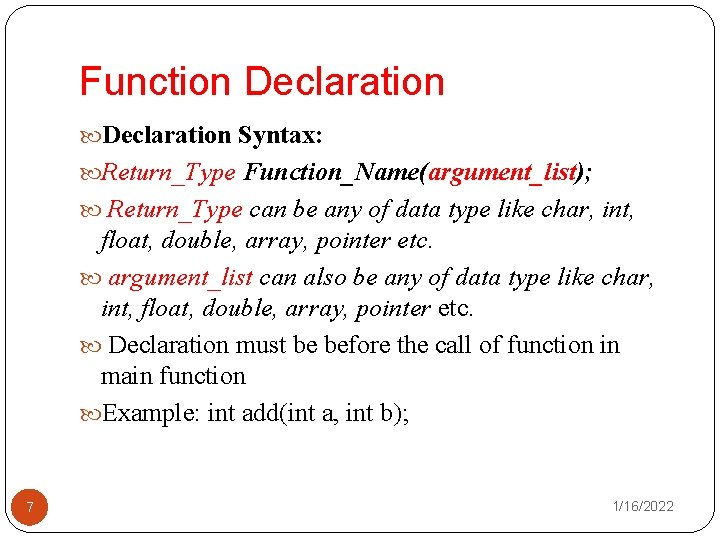Function Declaration Syntax: Return_Type Function_Name(argument_list); Return_Type can be any of data type like char, int, float, double, array, pointer etc. argument_list can also be any of data type like char, int, float, double, array, pointer etc. Declaration must be before the call of function in main function Example: int add(int a, int b); 7 1/16/2022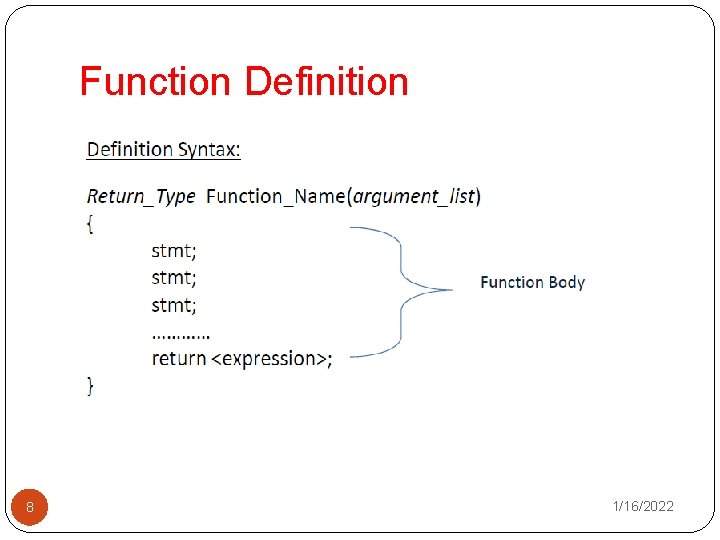Function Definition 8 1/16/2022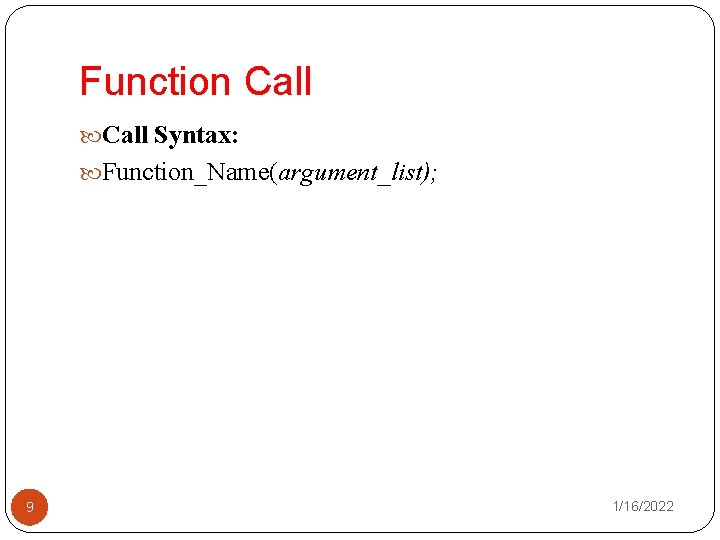Function Call Syntax: Function_Name(argument_list); 9 1/16/2022Sample Example #include<stdio. h> int sum (int, int); //function declaration void main(){ int p; p=sum(3, 4); //function call printf(“%d”, p); } int sum( int a, int b){ int s; //function body s=a+b; return s; //function returning a value 10} 1/16/2022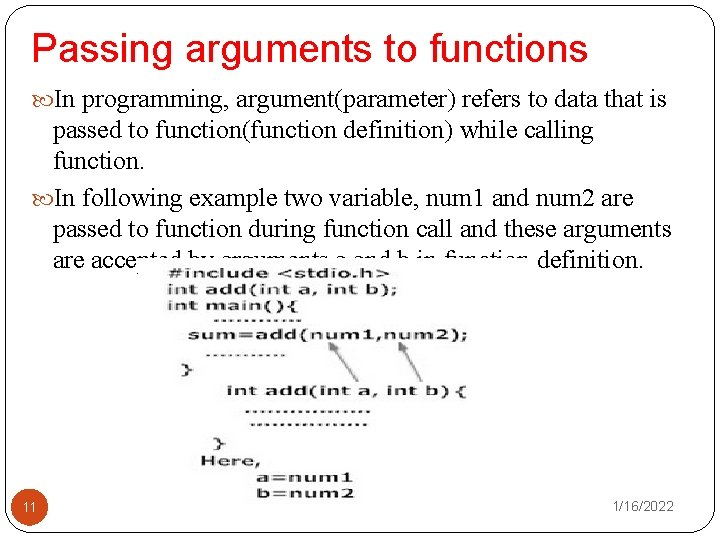Passing arguments to functions In programming, argument(parameter) refers to data that is passed to function(function definition) while calling function. In following example two variable, num 1 and num 2 are passed to function during function call and these arguments are accepted by arguments a and b in function definition. 11 1/16/2022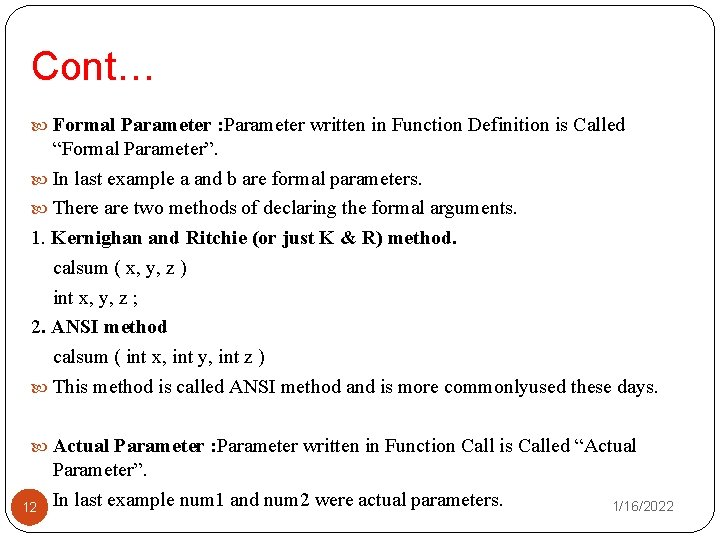Cont… Formal Parameter : Parameter written in Function Definition is Called “Formal Parameter”. In last example a and b are formal parameters. There are two methods of declaring the formal arguments. 1. Kernighan and Ritchie (or just K & R) method. calsum ( x, y, z ) int x, y, z ; 2. ANSI method calsum ( int x, int y, int z ) This method is called ANSI method and is more commonlyused these days. Actual Parameter : Parameter written in Function Call is Called “Actual Parameter”. 12 In last example num 1 and num 2 were actual parameters. 1/16/2022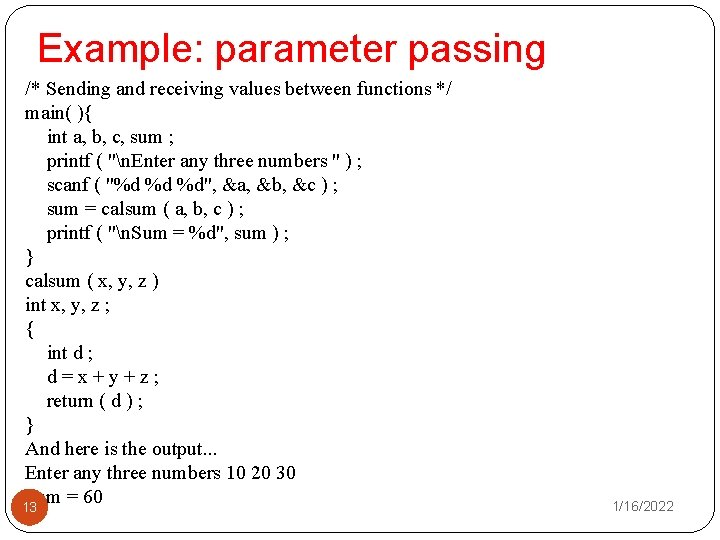Example: parameter passing /* Sending and receiving values between functions */ main( ){ int a, b, c, sum ; printf ( "n. Enter any three numbers " ) ; scanf ( "%d %d %d", &a, &b, &c ) ; sum = calsum ( a, b, c ) ; printf ( "n. Sum = %d", sum ) ; } calsum ( x, y, z ) int x, y, z ; { int d ; d=x+y+z; return ( d ) ; } And here is the output. . . Enter any three numbers 10 20 30 Sum = 60 13 1/16/2022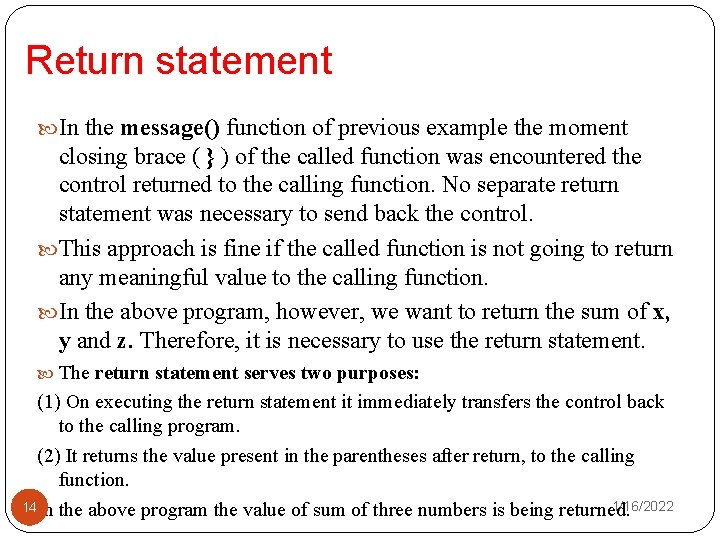Return statement In the message() function of previous example the moment closing brace ( } ) of the called function was encountered the control returned to the calling function. No separate return statement was necessary to send back the control. This approach is fine if the called function is not going to return any meaningful value to the calling function. In the above program, however, we want to return the sum of x, y and z. Therefore, it is necessary to use the return statement. The return statement serves two purposes: (1) On executing the return statement it immediately transfers the control back to the calling program. (2) It returns the value present in the parentheses after return, to the calling function. 1/16/2022 14 In the above program the value of sum of three numbers is being returned.Return statement(Cont…) There is no restriction on the number of return statements that may be present in a function. Also, the return statement need not always be present at the end of the called function. The following program illustrates these facts. fun( ){ char ch ; printf ( "n. Enter any alphabet " ) ; scanf ( "%c", &ch ) ; if ( ch >= 65 && ch <= 90 ) return ( ch ) ; else return ( ch + 32 ) ; } In this function different return statements will be executed depending on whether ch is capital or not. 15 1/16/2022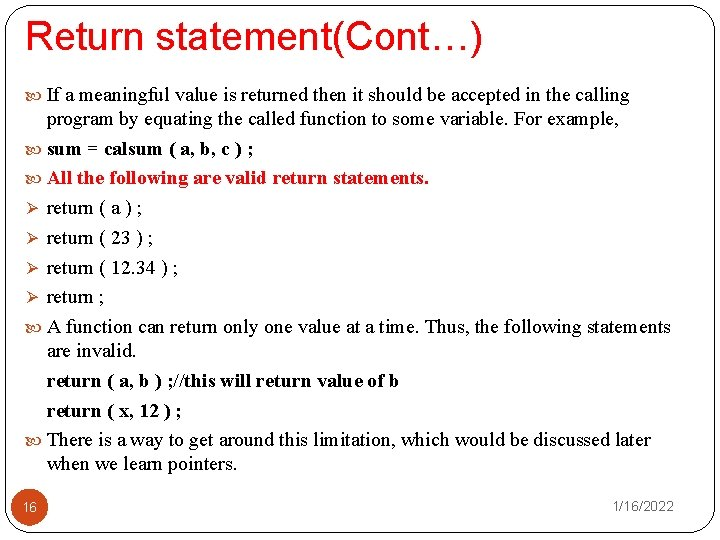Return statement(Cont…) If a meaningful value is returned then it should be accepted in the calling program by equating the called function to some variable. For example, sum = calsum ( a, b, c ) ; All the following are valid return statements. Ø return ( a ) ; Ø return ( 23 ) ; Ø return ( 12. 34 ) ; Ø return ; A function can return only one value at a time. Thus, the following statements are invalid. return ( a, b ) ; //this will return value of b return ( x, 12 ) ; There is a way to get around this limitation, which would be discussed later when we learn pointers. 16 1/16/2022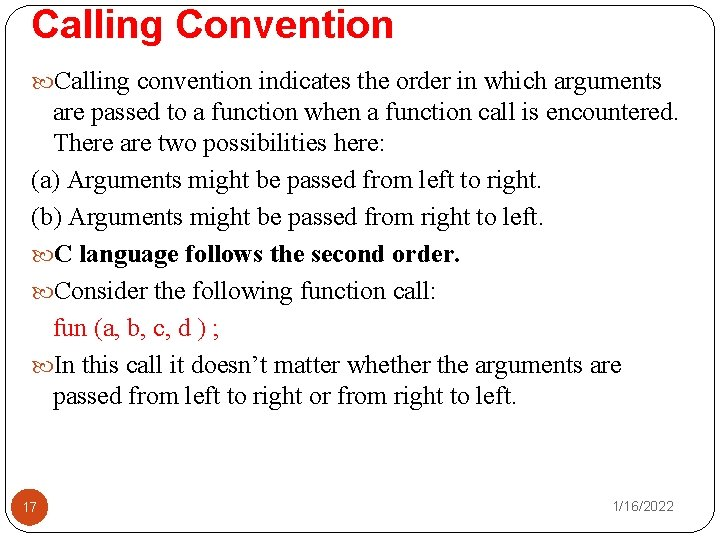Calling Convention Calling convention indicates the order in which arguments are passed to a function when a function call is encountered. There are two possibilities here: (a) Arguments might be passed from left to right. (b) Arguments might be passed from right to left. C language follows the second order. Consider the following function call: fun (a, b, c, d ) ; In this call it doesn’t matter whether the arguments are passed from left to right or from right to left. 17 1/16/2022Calling Convention(Cont…) • However, in some function call the order of passing arguments becomes an important consideration. • For example: 18 1/16/2022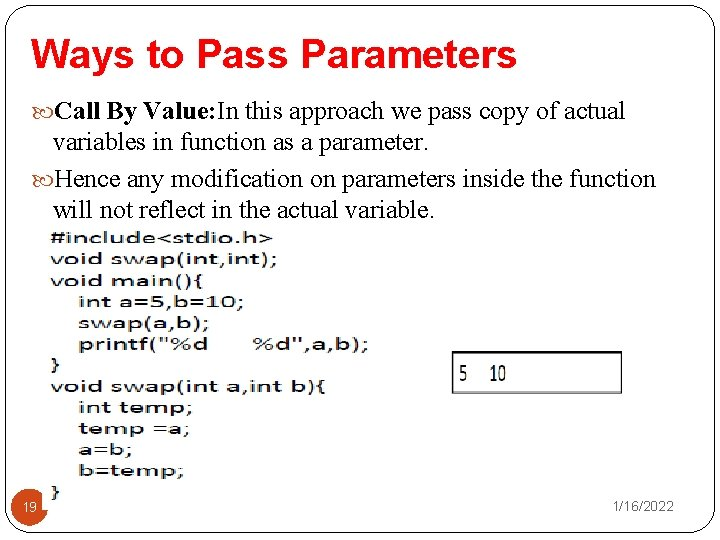Ways to Pass Parameters Call By Value: In this approach we pass copy of actual variables in function as a parameter. Hence any modification on parameters inside the function will not reflect in the actual variable. 19 1/16/2022Ways to Pass Parameters(Cont. . ) Call By Reference: In this approach we pass memory address of actual variables in function as a parameter. Hence any modification on parameters inside the function will reflect in the actual variable. 20 1/16/2022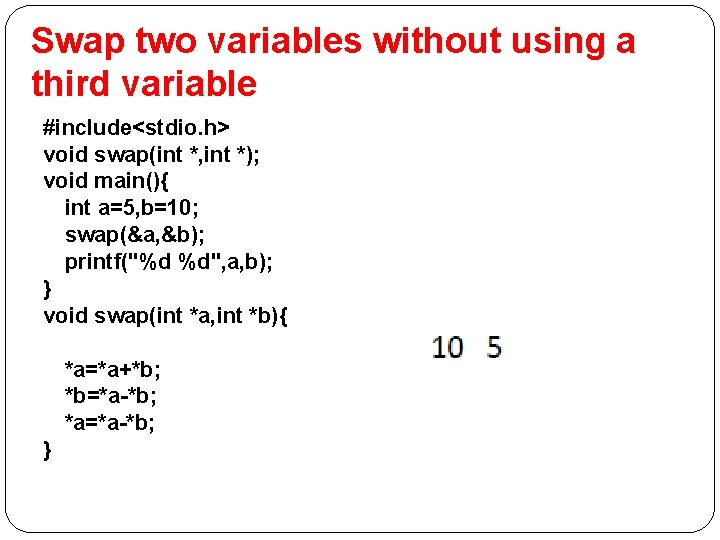Swap two variables without using a third variable #include<stdio. h> void swap(int *, int *); void main(){ int a=5, b=10; swap(&a, &b); printf("%d %d", a, b); } void swap(int *a, int *b){ *a=*a+*b; *b=*a-*b; *a=*a-*b; }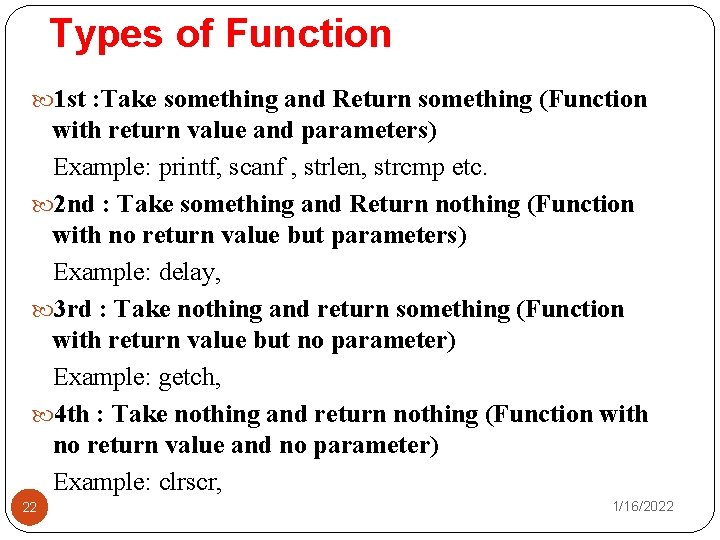Types of Function 1 st : Take something and Return something (Function with return value and parameters) Example: printf, scanf , strlen, strcmp etc. 2 nd : Take something and Return nothing (Function with no return value but parameters) Example: delay, 3 rd : Take nothing and return something (Function with return value but no parameter) Example: getch, 4 th : Take nothing and return nothing (Function with no return value and no parameter) Example: clrscr, 22 1/16/2022Take something and Return something 23 1/16/2022Take something and Return nothing 24 1/16/2022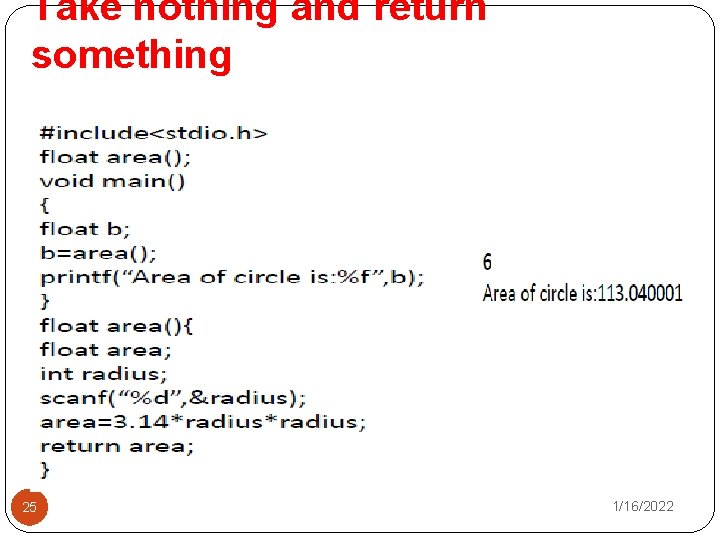Take nothing and return something 25 1/16/2022Take nothing and return nothing 26 1/16/2022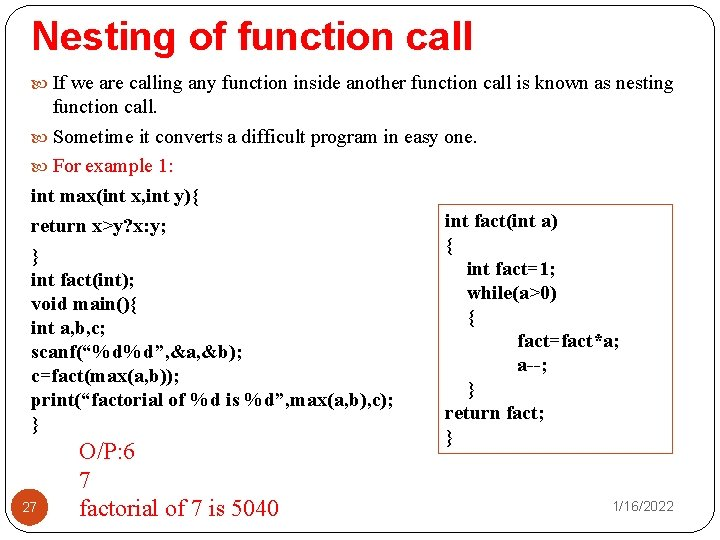Nesting of function call If we are calling any function inside another function call is known as nesting function call. Sometime it converts a difficult program in easy one. For example 1: int max(int x, int y){ int fact(int a) return x>y? x: y; { } int fact=1; int fact(int); while(a>0) void main(){ { int a, b, c; fact=fact*a; scanf(“%d%d”, &a, &b); a--; c=fact(max(a, b)); } print(“factorial of %d is %d”, max(a, b), c); return fact; } } 27 O/P: 6 7 factorial of 7 is 5040 1/16/2022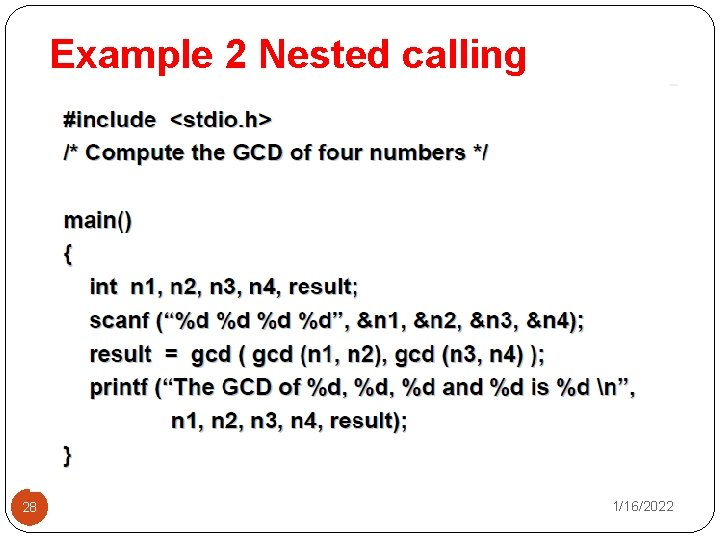Example 2 Nested calling 28 1/16/2022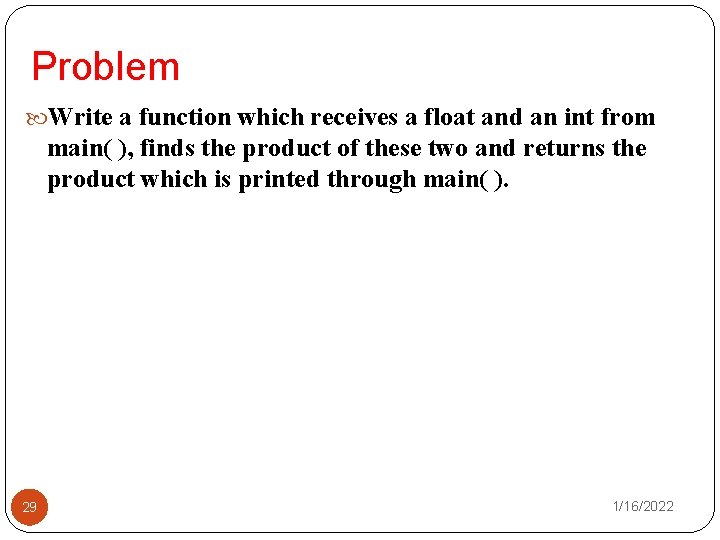Problem Write a function which receives a float and an int from main( ), finds the product of these two and returns the product which is printed through main( ). 29 1/16/2022